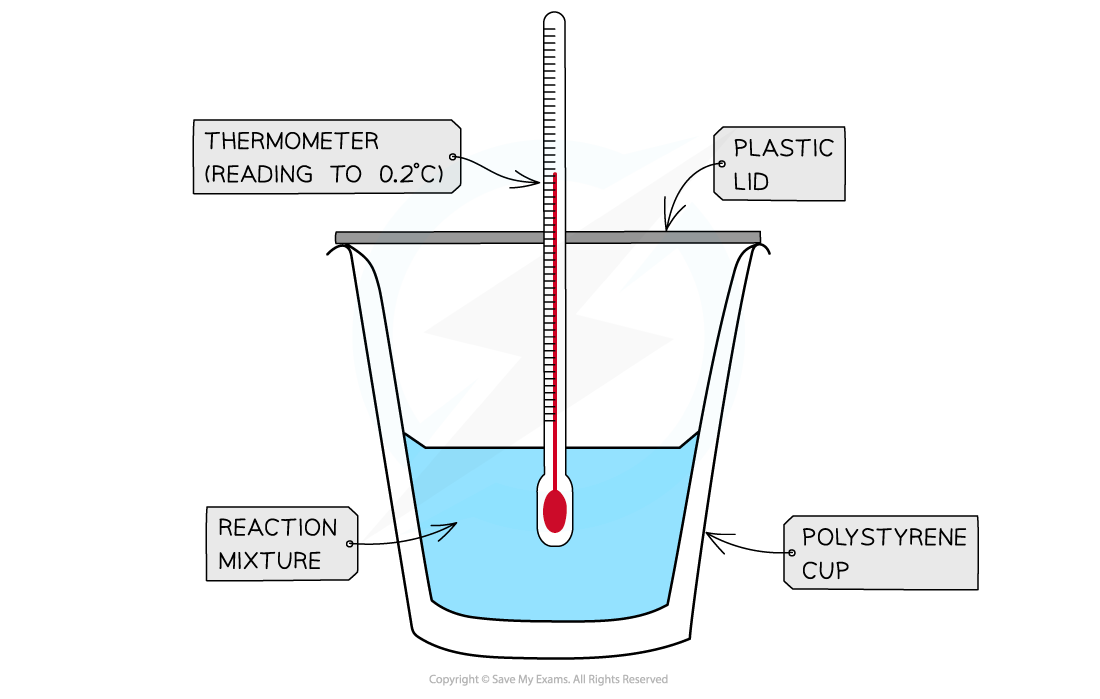# IB DP Chemistry: SL复习笔记5.1.3 Calorimetry

### Calorimetry

#### Measuring enthalpy changes

• Calorimetry is a technique used to measure changes in enthalpy of chemical reactions
• calorimeter can be made up of a polystyrene drinking cup, a vacuum flask or metal canA polystyrene cup can act as a calorimeter to find enthalpy changes in a chemical reaction

• The energy needed to raise the temperature of 1 g of a substance by 1 K is called the specific heat capacity (c) of the liquid
• The specific heat capacity of water is 4.18 J g-1 K-1
• The energy transferred as heat can be calculated by:Equation for calculating energy transferred in a calorimeter

#### Worked Example

The energy from 0.01 mol of propan-1-ol was used to heat up 250 g of water. The temperature of the water rose from 298K to 310K (the specific heat capacity of water is 4.18 J g-1 K-1.Calculate the enthalpy of combustion.

Step 1: q = m x c x ΔT

(of water) = 250 g

(of water) = 4.18 J g-1 K-1

Δ(of water) = 310 - 298 K = 12 K

Step 2: q = 250 x 4.18 x 12= 12 540 J

Step 3:  This is the energy released by 0.01 mol of propan-1-ol

Total energy    ΔH = q ÷ n = 12 540 J ÷ 0.01 mol = 1 254 000 J mol-1

Total energy = - 1254 kJ mol-1

#### Exam Tip

There's no need to convert the temperature units in calorimetry as the change in temperature in oC is equal to the change in temperature in K